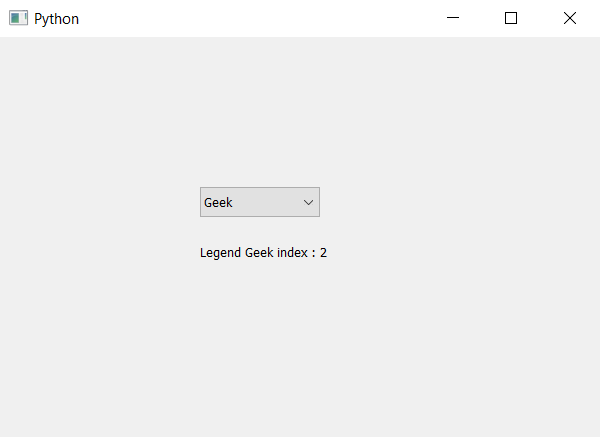Related Articles

# PyQt5 – Finding the item through text in ComboBox

• Last Updated : 22 Apr, 2020

In this article we will see how we can find the item index with the help of text in it. We know we can add items with the help of `addItem` and `addItems` method, in order to find the item with the help of `findText` method.

Syntax : combo_box.findText(text)

Argument : It takes string as argument.

Return : It return integer i.e index of text if text doesn’t exist it return -1

Below is the implementation –

 `# importing libraries``from` `PyQt5.QtWidgets ``import` `*` `from` `PyQt5 ``import` `QtCore, QtGui``from` `PyQt5.QtGui ``import` `*` `from` `PyQt5.QtCore ``import` `*` `import` `sys`` ` ` ` `class` `Window(QMainWindow):`` ` `    ``def` `__init__(``self``):``        ``super``().__init__()`` ` `        ``# setting title``        ``self``.setWindowTitle(``"Python "``)`` ` `        ``# setting geometry``        ``self``.setGeometry(``100``, ``100``, ``600``, ``400``)`` ` `        ``# calling method``        ``self``.UiComponents()`` ` `        ``# showing all the widgets``        ``self``.show()`` ` `    ``# method for widgets``    ``def` `UiComponents(``self``):`` ` `        ``# creating a combo box widget``        ``self``.combo_box ``=` `QComboBox(``self``)`` ` `        ``# setting geometry of combo box``        ``self``.combo_box.setGeometry(``200``, ``150``, ``120``, ``30``)`` ` `        ``# geek list``        ``geek_list ``=` `[``"Geek"``, ``"Geeky Geek"``, ``"Legend Geek"``, ``"Ultra Legend Geek"``]`` ` `        ``# adding list of items to combo box``        ``self``.combo_box.addItems(geek_list)`` ` `        ``# text``        ``text ``=` `"Legend Geek"`` ` `        ``# finding index of text``        ``index ``=` `self``.combo_box.findText(text)`` ` `        ``# label to show index``        ``label ``=` `QLabel(text ``+` `" index : "` `+` `str``(index), ``self``)`` ` `        ``# setting geometry of label``        ``label.setGeometry(``200``, ``200``, ``200``, ``30``)`` ` `# create pyqt5 app``App ``=` `QApplication(sys.argv)`` ` `# create the instance of our Window``window ``=` `Window()`` ` `# start the app``sys.exit(App.``exec``())`

Output :Attention geek! Strengthen your foundations with the Python Programming Foundation Course and learn the basics.

To begin with, your interview preparations Enhance your Data Structures concepts with the Python DS Course. And to begin with your Machine Learning Journey, join the Machine Learning – Basic Level Course

My Personal Notes arrow_drop_up